Home    |    Teacher    |    Parents    |    Glossary    |    About UsExponents are a shorthand way to show how many times a number, called the base, is multiplied times itself. A number with an exponent is said to be "raised to the power" of that exponent.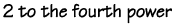Any number raised to the zero power (except 0) equals 1. Any number raised to the power of one equals itself.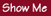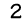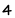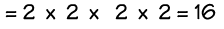If two numbers with exponents have the same base, you can multiply them by adding the exponents.Homework Help | Pre-Algebra | NumbersEmail this page to a friendSearch·  Place value·  Decimal numbers·  Estimating and     rounding·  Adding / subtracting     decimals·  Multiplying decimals·  Dividing decimals·  Percent·  Exponents·  Square roots·  Signed integers·  Adding and     subtracting integers·  Multiplying and     dividing integers·  Properties of integers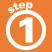First Glance In Depth Examples WorkoutExponents